SSC CGL Previous Year Questions: Geometry- 5

# SSC CGL Previous Year Questions: Geometry- 5 - SSC CGL

Test Description

## 40 Questions MCQ Test SSC CGL (Tier - 1) - Previous Year Papers (Topic Wise) - SSC CGL Previous Year Questions: Geometry- 5

SSC CGL Previous Year Questions: Geometry- 5 for SSC CGL 2023 is part of SSC CGL (Tier - 1) - Previous Year Papers (Topic Wise) preparation. The SSC CGL Previous Year Questions: Geometry- 5 questions and answers have been prepared according to the SSC CGL exam syllabus.The SSC CGL Previous Year Questions: Geometry- 5 MCQs are made for SSC CGL 2023 Exam. Find important definitions, questions, notes, meanings, examples, exercises, MCQs and online tests for SSC CGL Previous Year Questions: Geometry- 5 below.
Solutions of SSC CGL Previous Year Questions: Geometry- 5 questions in English are available as part of our SSC CGL (Tier - 1) - Previous Year Papers (Topic Wise) for SSC CGL & SSC CGL Previous Year Questions: Geometry- 5 solutions in Hindi for SSC CGL (Tier - 1) - Previous Year Papers (Topic Wise) course. Download more important topics, notes, lectures and mock test series for SSC CGL Exam by signing up for free. Attempt SSC CGL Previous Year Questions: Geometry- 5 | 40 questions in 40 minutes | Mock test for SSC CGL preparation | Free important questions MCQ to study SSC CGL (Tier - 1) - Previous Year Papers (Topic Wise) for SSC CGL Exam | Download free PDF with solutions
 1 Crore+ students have signed up on EduRev. Have you?
SSC CGL Previous Year Questions: Geometry- 5 - Question 1

### AB is the chord of a circle with centre O and DOC is a line segment originating from a point D on the circle and intersecting, AB produced at C such that BC = OD. If ∠BCD = 20°, then ∠AOD =?   (SSC CGL 2nd Sit. 2013)

Detailed Solution for SSC CGL Previous Year Questions: Geometry- 5 - Question 1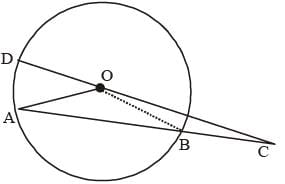Here BC = OD = radius {given}
In ΔBOC,
∴ ∠BOC = ∠BCO = 20°
∠OBA = ∠BOC + ∠BCO = 20° + 20° = 40°
Again, In DAOB,
∠OAB = ∠OBA = 40°
∴ ∠AOB = 180° – 40° – 40° = 100°
Again,
∠AOD + ∠AOB + ∠BOC = 180°
∠AOD + 100° + 20° = 180°
∠AOD = 180° – 120° = 60°

SSC CGL Previous Year Questions: Geometry- 5 - Question 2

### ABC is a triangle. The bisectors of the internal angle ∠B and external angle ∠C intersect at D. If ∠BDC = 50°, then ∠A is   (SSC CGL 2nd Sit.  2013)

Detailed Solution for SSC CGL Previous Year Questions: Geometry- 5 - Question 2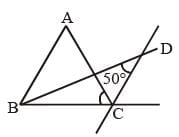From question ∠BDC = 50°
In ΔBDC, ∠DBC + ∠BDC + ∠BCD = 180°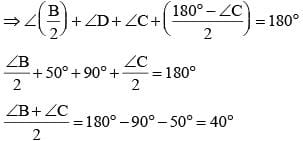∠B + ∠C = 40 × 2 = 80°
From ΔABC, ∠A + ∠B + ∠C = 180°
∠A + 80° = 180°
∠A = 180° – 80° = 100°

SSC CGL Previous Year Questions: Geometry- 5 - Question 3

### ABCD is a cyclic trapezium with AB || DC and AB = diameter of the circle. If ∠CAB = 30° then ∠ADC is   (SSC CGL 2nd Sit.  2013)

Detailed Solution for SSC CGL Previous Year Questions: Geometry- 5 - Question 3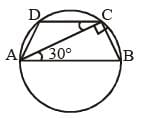AB is a diameter of the circle
∴ ∠ACB = 90° {Angle made by the diameter on the semicircle
∠BAC = 30° {given)
∴ ∠BAC = ∠ACD = 30°   {As AB || CD}.
∠BCD = 90° + 30° = 120°
∴ ∠BAD + ∠BCD = 180°
∠BAD = 180° – 120° = 60°
∴ ∠ADC = 180° – 60° = 120°.

SSC CGL Previous Year Questions: Geometry- 5 - Question 4

ABC is an isosceles triangle such that AB = AC and ∠B = 35°. AD is the median to the base BC. Then ∠BAD is:    (SSC CGL 1st Sit. 2013)

Detailed Solution for SSC CGL Previous Year Questions: Geometry- 5 - Question 4

As, AB = AC
∴ ∠ABC =  ∠ACB = 35°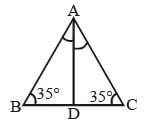∠BAC = 180° – 35° – 35° = 110°

SSC CGL Previous Year Questions: Geometry- 5 - Question 5

AB and CD are two parallel chords of a circle such that AB = 10 cm and CD = 24 cm. If the chords are on the opposite sides of the centre and distance between them is 17 cm, then the radius of the circle is:   (SSC CGL 1st Sit. 2013)

Detailed Solution for SSC CGL Previous Year Questions: Geometry- 5 - Question 5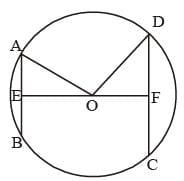AB = 10 cm, AE = 5 cm
OE = x
CD = 24 cm, DF = 12 cm
OF = 17 – x
⇒ 52 + x2 = 122 + (17 – x)2
⇒ 25 + x2 = 144 + 289 – 34x + x2
⇒ 34x = 408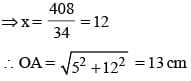SSC CGL Previous Year Questions: Geometry- 5 - Question 6

P and Q are two points on a circle with centre at O. R is a point on the minor arc of the circle, between the points P and Q. The tangents to the circle at the points P and Q meet each other at the point S. If ∠PSQ = 20°, ∠PRQ =?   (SSC CGL 1st Sit. 2013)

Detailed Solution for SSC CGL Previous Year Questions: Geometry- 5 - Question 6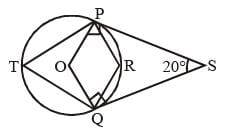In quadrilateral POQS, ∠S = 20°
∠OPS = ∠OQS = 90°, ∠POQ = ?.
{Since PS and QS are tangent to the circle}
∴ Sum of angles in a quadrilateral = 360°
∠OPS + ∠S + ∠OQS + ∠POQ = 360°
90° + 20° + 90° + ∠POQ = 360°
⇒ ∠POQ = 160°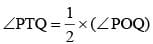{Angle made on perimeter by the same chord is half of the angle made at the centre}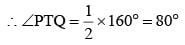∠PTQ + ∠PRS = 180°
80° + ∠PRQ = 180°  ⇒ ∠PRQ = 180° – 80° = 100°

SSC CGL Previous Year Questions: Geometry- 5 - Question 7

If ABCD be a rectangle and P, Q, R, S be the mid points of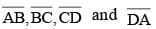respectively, then the area of the quadrilateral PQRS is equal to: (SSC CGL 1st Sit. 2013)

Detailed Solution for SSC CGL Previous Year Questions: Geometry- 5 - Question 7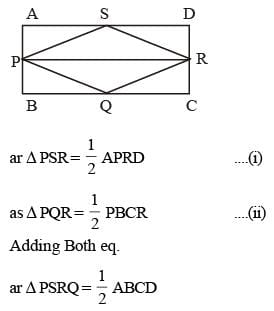SSC CGL Previous Year Questions: Geometry- 5 - Question 8

A chord of length 30 cm is at a distance of 8 cm from the centre of a circle. The radius of the circle is:    (SSC CGL 1st Sit. 2013)

Detailed Solution for SSC CGL Previous Year Questions: Geometry- 5 - Question 8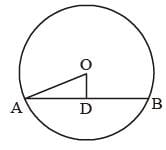Here chord AB = 300 cm
OD = 8 cm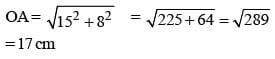SSC CGL Previous Year Questions: Geometry- 5 - Question 9

In a triangle ABC, AB = AC, ∠BAC = 40°. Then the external angle at B is:    (SSC CGL 1st Sit. 2013)

Detailed Solution for SSC CGL Previous Year Questions: Geometry- 5 - Question 9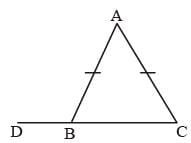Since, AB = AC {given}
∠ABC = ∠ACB
∠BAC = 40°  {given}
∴ ∠ABC + ∠ACB = {180° – 40°} = 140°
∴ ∠ABC  = 70°
​​​​​​​∴ ∠ABD = 180° – 70° = 110°

SSC CGL Previous Year Questions: Geometry- 5 - Question 10

A chord AB of a circle C1 of radius (√3 + 1) cm touches a circle C2 which is concentric to C1. If the radius of C2 is (√3 - 1) cm, the length of AB is:    (SSC CGL 1st Sit. 2013)

Detailed Solution for SSC CGL Previous Year Questions: Geometry- 5 - Question 10

Let A chord AB of circle C1, touches the concentric circle C2 at point ‘P’.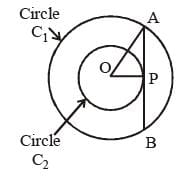Here OA = radius of circle C1 = (√3 + 1)
OP = radius of circle C2 = (√3 - 1)
As AP is a tangent to the circle C2.
∴ ∠OPA = 90°
Now, from ΔOPA, (OA)2 = (OP)2 + (AP)2
(AP)2 = (OA)2 – (OP)2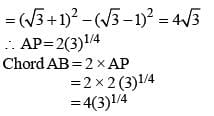SSC CGL Previous Year Questions: Geometry- 5 - Question 11

If ΔABC is similar to ΔDEF such that BC = 3 cm, EF = 4 cm and area of ΔABC = 54 cm2, then the area of ΔDEF is:    (SSC CGL 1st Sit. 2013)

Detailed Solution for SSC CGL Previous Year Questions: Geometry- 5 - Question 11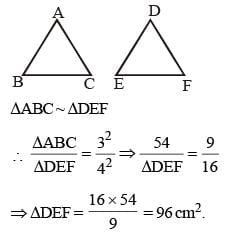SSC CGL Previous Year Questions: Geometry- 5 - Question 12

The perpendiculars, drawn from the vertices to the opposite sides of a triangle, meet at the point whose name is    (SSC CHSL 2013)

Detailed Solution for SSC CGL Previous Year Questions: Geometry- 5 - Question 12

Orthocenter is the point where all three altitudes of the triangle intersect. An altitude is a line which passes through a vertex of the triangle and is perpendicular to the opposite side.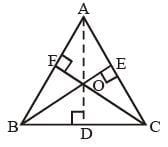Here in the triangle ABC, AD, BE and CF are three altitudes drawn from point A, B and C on the side BC, AC and AB respectively. All the three altitudes intersect each other at a common point ‘O’. That point ‘O’ is called ‘Orthocenter’ of the triangle ABC.

SSC CGL Previous Year Questions: Geometry- 5 - Question 13

If in ΔABC, ∠ABC = 5∠ACB and ∠BAC = 3 ∠ACB, then ∠ABC =    (SSC CHSL 2013)

Detailed Solution for SSC CGL Previous Year Questions: Geometry- 5 - Question 13

∠A + ∠B + ∠C = 180°
3∠C + 5∠C + ∠C = 180°
9∠C = 180°
∠C = 20°
∠B = 100°

SSC CGL Previous Year Questions: Geometry- 5 - Question 14

360 sq. cm and 250 sq. cm are the areas of two similar triangles. If the length of one of the sides of the first triangle be 8 cm, then the length of the corresponding side of the second triangle is    (SSC CHSL 2013)

Detailed Solution for SSC CGL Previous Year Questions: Geometry- 5 - Question 14

Let the length of the corresponding side of other triangle is x. Then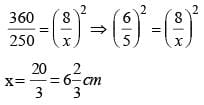SSC CGL Previous Year Questions: Geometry- 5 - Question 15

A chord 12 cm long is drawn in a circle of diameter 20 cm. The distance of the chord from the centre is    (SSC CHSL 2013)

Detailed Solution for SSC CGL Previous Year Questions: Geometry- 5 - Question 15

Given, AB = 12 cm; CD = 20 cm
OE = ?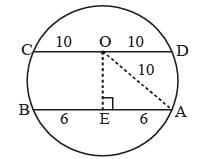Now, AE = EB = 6cm (The line drawn from centre of circle to the chord bisect the chord)
In ΔOAE, By Pythagoras theorem
(OA)2 = (OE)2 + (AE)2 ⇒ (10)2 = (OE)2 + (6)2
100 – 36 = (OE)2 ⇒ 64 = OE2 ⇒ OE = 8 cm

SSC CGL Previous Year Questions: Geometry- 5 - Question 16

From a point P, two tangents PA and PB are drawn to a circle with centre O. If OP is equal to diameter of the circle, then ∠APB is    (SSC CHSL 2013)

Detailed Solution for SSC CGL Previous Year Questions: Geometry- 5 - Question 16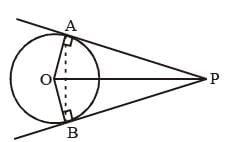AP and PB are two tangents to the circle
∴ ∠OAP = ∠OBP = 90°
In ΔOAP, Let ∠OPA = θ.
OP = 2 × radius {given}
∴ OP = 2 × OA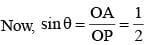∴ sinθ = sin 30° ⇒ θ = 30°
Again In ΔBOP, ∠OPA = ∠OPB = θ = 30° {By symmetry}
∴ ∠APB = 30° + 30° = 60°.

SSC CGL Previous Year Questions: Geometry- 5 - Question 17

In ΔABC. ∠A + ∠B = 145° and ∠C + 2∠B = 180°. State which one of the following relations is true? (SSC Sub. Ins. 2013)

Detailed Solution for SSC CGL Previous Year Questions: Geometry- 5 - Question 17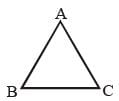∠A + ∠B = 145°
∠C + 180° – 145° = 35°
∠C + 2∠B = 180°
⇒ 2∠B = 180° – 35° = 145°
⇒ ∠B = 145/2 = 72.5 ° = ∠A
∠B > ∠C
∴ CA > AB

SSC CGL Previous Year Questions: Geometry- 5 - Question 18

In a triangle ABC, BC is produced to D so that CD = AC. If ∠BAD = 111° and ∠ACB = 80°, then the measure of ∠ABC is:   (SSC Sub. Ins. 2013)

Detailed Solution for SSC CGL Previous Year Questions: Geometry- 5 - Question 18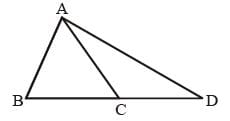∠ACB = 80°
∠ACD = 180° – 80° = 100°
= 80/2 = 40°
∠BAC = 111° – 40°
= 71°
∠ABC = 180° – 71° – 80° = 29°

SSC CGL Previous Year Questions: Geometry- 5 - Question 19

The areas of two similar triangles ABC and DEF are 20 cm2 and 45 cm2 respectively. If AB = 5 cm. then DE is equal to:   (SSC Sub. Ins. 2013)

Detailed Solution for SSC CGL Previous Year Questions: Geometry- 5 - Question 19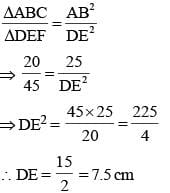SSC CGL Previous Year Questions: Geometry- 5 - Question 20

In the following figure. AB be diameter of a circle whose centre is O. If  ∠AOE = 150°. ∠DAO = 51° then the measure of ∠CBE is:    (SSC Sub. Ins. 2013)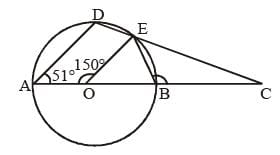Detailed Solution for SSC CGL Previous Year Questions: Geometry- 5 - Question 20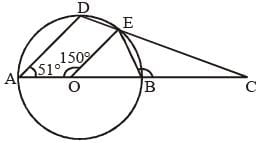∠AOE = 150°
∠DAO = 51°
∠EOB = 180° – 150° = 30°
∴ ∠OEB = ∠OBE =  150/2 = 75°
∴ ∠CBE = 180° – 75° = 105°

SSC CGL Previous Year Questions: Geometry- 5 - Question 21

Triangle PQR circumscribes a circle with centre O and radius r cm such that ∠PQR = 90°. If PQ = 3 cm, QR= 4 cm, then the value of r is:    (SSC Sub. Ins. 2013)

Detailed Solution for SSC CGL Previous Year Questions: Geometry- 5 - Question 21

PR2 = PQ2 + PR2 = 32 + 42 = 25
∴ PR = √25 = 5 cm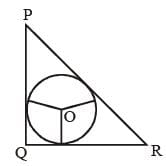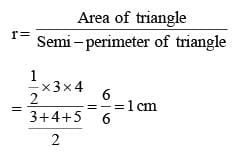SSC CGL Previous Year Questions: Geometry- 5 - Question 22

If area of an equilateral triangle is a and height b, then value of  b2/a  is:   (SSC Sub. Ins. 2013)

Detailed Solution for SSC CGL Previous Year Questions: Geometry- 5 - Question 22

Let side of triangle = x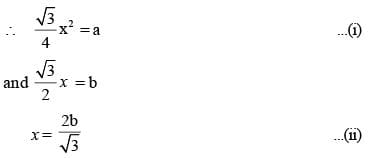Putting x in equation (i)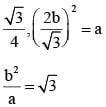SSC CGL Previous Year Questions: Geometry- 5 - Question 23

A wheel rotates 3.5 times in one second. What time (in seconds) does the wheel take to rotate 55 radian of angle?   (SSC CGL 2nd Sit. 2012)

Detailed Solution for SSC CGL Previous Year Questions: Geometry- 5 - Question 23

Radian covered in one second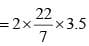Time required to covered 55 radian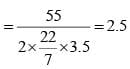SSC CGL Previous Year Questions: Geometry- 5 - Question 24

A and B are centres of the two circles whose radii are 5 cm and 2 cm respectively. The direct common tangents to the circles meet AB extended at P. Then P divides AB.   (SSC CGL 2nd Sit. 2012)

Detailed Solution for SSC CGL Previous Year Questions: Geometry- 5 - Question 24Here RQ is the common tangent which touches circles with centre A and B at point R and Q respectively
∴ ∠ARQ = ∠BQR = 90°
On extending the line AB, tangent RQ meet the line AB at point P.
Now, In DPBQ and DPAR,
BQ || AR, ∠P = ∠P, ∠Q = ∠R  ⇒ ∠A = ∠B.
thus, DPBQ ~ DPAR {from AA theorem}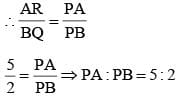Hence, point P, divides line AB into 5 : 2 ratio externally.

SSC CGL Previous Year Questions: Geometry- 5 - Question 25

O is the centre of the circle passing through the points A, B and C such that ∠BAO = 30°, ∠BCO = 40° and ∠AOC = x°.
What is the value of x?   (SSC CGL 2nd Sit. 2012)

Detailed Solution for SSC CGL Previous Year Questions: Geometry- 5 - Question 25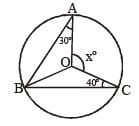In ΔAOB
AO = BO (radii of circles)
∴ ∠ABO = ∠BAO = 30º In DBOC
BO = CO (radii of circles)
∴ ∠BCO = ∠OBC = 40º
∠ABC = ∠ABO + ∠OBC
∠ABC = 30° + 40° = 70°
2 × ∠ABC = ∠AOC ⇒ x° = 140

SSC CGL Previous Year Questions: Geometry- 5 - Question 26

Two circles intersect each other at P and Q. PA and PB are two diameters. Then ∠AQB is   (SSC CGL 2nd Sit. 2012)

Detailed Solution for SSC CGL Previous Year Questions: Geometry- 5 - Question 26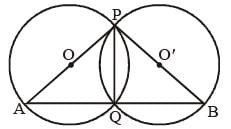Let O and O' be the centre of two intersecting circle, where point of intersection are P and Q and PA and PB are their diameter respectively.
∠AQP = 90° and ∠BQP = 90°
{∵ Angle in a semicircle is a right angle}
∠AQP + ∠BQP = 180°
∴ ∠AQB = 180º

SSC CGL Previous Year Questions: Geometry- 5 - Question 27

The bisector of ∠A of ΔABC cuts BC at D and the circumcircle of the triangle at E. Then   (SSC CGL 2nd Sit. 2012)

Detailed Solution for SSC CGL Previous Year Questions: Geometry- 5 - Question 27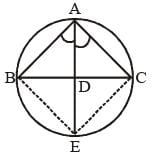In ΔABC, D is the mid-point of side BC, since, AD divide angle A.
∴ BD = DC
and ∠ABC = ∠AEC {angle in same sector of circle}
and ∠CAE = ∠CBE
from ΔABD and ΔACE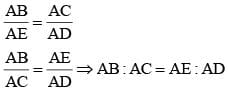SSC CGL Previous Year Questions: Geometry- 5 - Question 28

Two circles with same radius r intersect each other and one passes through the centre of the other. Then the length of the common chord is    (SSC CGL 2nd Sit. 2012)

Detailed Solution for SSC CGL Previous Year Questions: Geometry- 5 - Question 28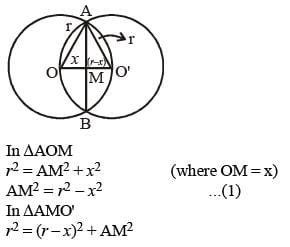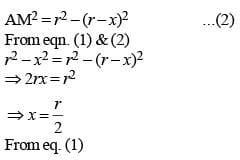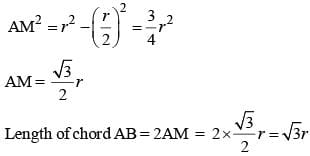SSC CGL Previous Year Questions: Geometry- 5 - Question 29

AB is a diameter of the circumcircle of ΔAPB; N is the foot of the perpendicular drawn from the point P on  AB. If AP = 8 cm and BP = 6 cm, then the length of BN is   (SSC CGL 2nd Sit. 2012)

Detailed Solution for SSC CGL Previous Year Questions: Geometry- 5 - Question 29

Since AB is a diameter. Then ∠APB = 90° (angle in the semicircle)
ΔBPN ~ ΔAPB
So, BN = BP2 / AB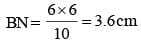SSC CGL Previous Year Questions: Geometry- 5 - Question 30

If P, R, T are the area of a parallelogram, a rhombus and a triangle standing on the same base and between the same parallels lines which of the following is true?   (SSC CGL 2nd Sit. 2012)

Detailed Solution for SSC CGL Previous Year Questions: Geometry- 5 - Question 30

Parallelogram Area = l × b
Rhombus Area = l × b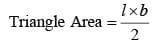Therefore R = P = 2T.

SSC CGL Previous Year Questions: Geometry- 5 - Question 31

ABC is a triangle. The medians CD and BE intersect each other at O. Then ΔODE : ΔABC is   (SSC CGL 2nd Sit. 2012)

Detailed Solution for SSC CGL Previous Year Questions: Geometry- 5 - Question 31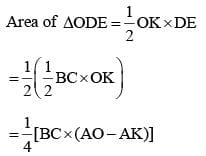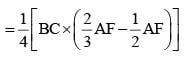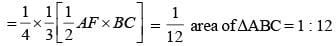SSC CGL Previous Year Questions: Geometry- 5 - Question 32

If D is the mid-point of the side BC of ΔABC and the area of ΔABD is 16 cm2, then the area of ΔABC is    (SSC CGL 2nd Sit. 2012)

Detailed Solution for SSC CGL Previous Year Questions: Geometry- 5 - Question 32

Area of ΔABD = 16 cm2
Area of ΔABC = 2 × Area of ΔABD [∵ In triangle, the midpoint of the opposite side, divides it into two congruent triangles. So their areas are equal and each is half the area of the original triangle]
⇒ 32 cm2

SSC CGL Previous Year Questions: Geometry- 5 - Question 33

The length of the circum-radius of a triangle having sides of lengths 12 cm, 16 cm and 20 cm is    (SSC CGL 2nd Sit. 2012)

Detailed Solution for SSC CGL Previous Year Questions: Geometry- 5 - Question 33

Let the sides a = 12 cm, b = 16cm, c = 20 cm then,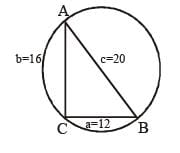there, a2 + b2 = c2
(12)2 + (16)2 = (20)2
144 + 256 = 400
400 = 400
∴ ΔABC is a right angle triangle, whose hypotenuse
AB = 20 cm.
As we know that the Length of the diameter of outer circle of right angle triangle is equal to its hypotenuse.
So, radius of required circle = 20/2 = 10 cm.

SSC CGL Previous Year Questions: Geometry- 5 - Question 34

The radius of the circumcircle of the triangle made by x-axis, y-axis and 4x + 3y = 12 is   (SSC CGL 2nd Sit. 2012)

Detailed Solution for SSC CGL Previous Year Questions: Geometry- 5 - Question 34

Putting x = 0 in 4x + 3y = 12 we get  y = 4
Putting y = 0 in 4x + 3y = 12 we get x = 3
The triangle so formed is right angle triangle with points (0, 0), (3, 0) and (0, 4)
So diameter is the hypotenus of triangle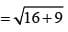= 5 unit

SSC CGL Previous Year Questions: Geometry- 5 - Question 35

A rail road curve is to be laid out on a circle. What radius should be used if the track is to change direction by 25° in a distance of 40 metres?   (SSC CGL 1st Sit. 2012)

Detailed Solution for SSC CGL Previous Year Questions: Geometry- 5 - Question 35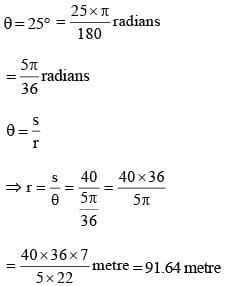SSC CGL Previous Year Questions: Geometry- 5 - Question 36

When a pendulum of length 50 cm oscillates, it produces an arc of 16 cm. The angle so formed in degree measure is (approx)   (SSC CGL 1st Sit. 2012)

Detailed Solution for SSC CGL Previous Year Questions: Geometry- 5 - Question 36

s = 16 cm
r = 50 cm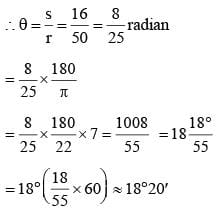SSC CGL Previous Year Questions: Geometry- 5 - Question 37

The external bisectors of ∠B and ∠C of ΔABC meet at point P. If ∠BAC = 80°, then ∠BPC is   (SSC CGL 1st Sit. 2012)

Detailed Solution for SSC CGL Previous Year Questions: Geometry- 5 - Question 37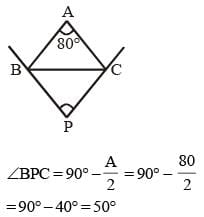SSC CGL Previous Year Questions: Geometry- 5 - Question 38

If I is the In-centre of ΔABC and ∠A = 60°, then the value of ∠BIC is   (SSC CGL 1st Sit. 2012)

Detailed Solution for SSC CGL Previous Year Questions: Geometry- 5 - Question 38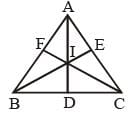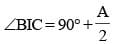= 90° + 30° = 120°

SSC CGL Previous Year Questions: Geometry- 5 - Question 39

Two circles with radii 5 cm and 8 cm touch each other externally at a point A. If a straight line through the point A cuts the circles at points P and Q respectively, then AP : AQ is   (SSC CGL 1st Sit. 2012)

Detailed Solution for SSC CGL Previous Year Questions: Geometry- 5 - Question 39∴ AP : AQ = 5 : 8

SSC CGL Previous Year Questions: Geometry- 5 - Question 40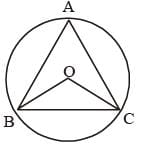BC is the chord of a circle with centre O. A is a point on major arc BC as shown in the above figure. What is the value of ∠BAC + ∠OBC?   (SSC CGL 1st Sit. 2012)

Detailed Solution for SSC CGL Previous Year Questions: Geometry- 5 - Question 40

∠BOC = 2∠BAC
∴ ∠OBC = ∠OCB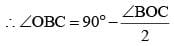= 90° – ∠BAC
∴ ∠BAC + ∠OBC
= 90° – ∠BAC + ∠BAC= 90°

## SSC CGL (Tier - 1) - Previous Year Papers (Topic Wise)

250 tests
Information about SSC CGL Previous Year Questions: Geometry- 5 Page
In this test you can find the Exam questions for SSC CGL Previous Year Questions: Geometry- 5 solved & explained in the simplest way possible. Besides giving Questions and answers for SSC CGL Previous Year Questions: Geometry- 5, EduRev gives you an ample number of Online tests for practice

250 tests